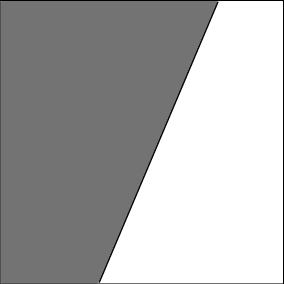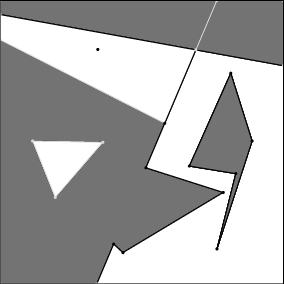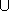# Chapter 162D Boolean Operations on Nef Polygons

Michael Seel

 16.1 Introduction 16.2 Construction and Composition 16.3 Exploration 16.4 Traits Classes 16.5 Implementation

## 16.1   Introduction

When working with polygonal and polyhedral sets, the mathematical model determines the kind of point set that can be represented. Nef polyhedra are the most general rectilinear polyhedral model.

Topological simpler models that are contained in the domain of Nef polyhedra are:

• convex polytopes normally defined as the convex hull of a nonempty finite set of points. Convex polytopes are compact closed and manifold sets.
• elementary polyhedra normally defined as the union of a finite number of convex polytopes.
• polyhedral sets nomally defined as the intersection of a finite number of closed halfspaces. Such sets are closed and convex but need not to be compact.
• linear polyhedra normally defined as the set of all points belonging to the simplices of a simplicial complex.

A planar Nef polyhedron is any set that can be obtained from a finite set of open halfspaces by set complement and set intersection operations. Due to the fact that all other binary set operations like union, difference and symmetric difference can be reduced to intersection and complement calculations, Nef polyhedra are also closed under those operations. Apart from the set complement operation there are more topological unary set operations that are closed in the domain of Nef polyhedra. Given a Nef polyhedron one can determine its interior, its boundary, and its closure, and also composed operations like regularization (defined to be the closure of the interior or a point set).Figure 16.1:  Two Nef polyhedra in the plane. A closed halfspace on the left and a complex polyhedron on the right. Note that the points on the squared boundary are at infinity.

## 16.2   Construction and Composition

Following the above definition, the data type Nef_polyhedron_2<T> allows construction of elementary Nef polyhedra and the binary and unary composition by the mentioned set operations.

In the following examples skip the typedefs at the beginning at first and take the types Point and Line to be models of the standard two-dimensional CGAL kernel (CGAL::Point_2<K> and CGAL::Line_2<K>). Their user interface is thus defined in the corresponding reference pages.

```File: examples/Nef_2/construction.cpp
```
```#include <CGAL/Gmpz.h>
#include <CGAL/Filtered_extended_homogeneous.h>
#include <CGAL/Nef_polyhedron_2.h>

typedef CGAL::Gmpz RT;
typedef CGAL::Filtered_extended_homogeneous<RT> Extended_kernel;
typedef CGAL::Nef_polyhedron_2<Extended_kernel> Nef_polyhedron;
typedef Nef_polyhedron::Point Point;
typedef Nef_polyhedron::Line  Line;

int main() {

Nef_polyhedron N1(Nef_polyhedron::COMPLETE);

Line l(2,4,2); // l : 2x + 4y + 2 = 0
Nef_polyhedron N2(l,Nef_polyhedron::INCLUDED);
Nef_polyhedron N3 = N2.complement();
CGAL_assertion(N1 == N2.join(N3));

Point p1(0,0), p2(10,10), p3(-20,15);
Point triangle = { p1, p2, p3 };
Nef_polyhedron N4(triangle, triangle+3);
Nef_polyhedron N5 = N2.intersection(N4);
CGAL_assertion(N5 <= N2 && N5 <= N4);

return 0;
}
```

Planar halfspaces (as used in the definition) are modelled by oriented lines. In the previous example N1 is the Nef polyhedron representing the full plane, N2 is the closed halfspace left of the oriented line with equation 2x + 4y + 2 = 0 including the line, N3 is the complement of N2 and therefore it must hold that N2N3 = N1.

Additionally one can construct Nef polyhedra from iterator ranges that hold simple polygonal chains. In the example N4 is the triangle spanned by the vertices (0,0), (10,10), (-20,15). Note that the construction from a simple polygonal chain has several cases and preconditions that are described in the reference manual page of Nef_polyhedron_2<T>. The operator<= in the last assertion is a subset-or-equal comparison of two polyhedra.

Nef polyhedra have input and output operators that allows one to output them via streams and read them from streams. Graphical output is currently possible to a CGAL::Window_stream. The output operation is defined in CGAL/IO/Nef_polyhedron_2_Window_stream.h. For an elaborate example see the demo programs in the directory demo/Nef_2.

## 16.3   Exploration

By recursively composing binary and unary operations one can end with a very complex rectilinear structure. To explore that structure there is a data type Nef_polyhedron_2<T>::Explorer that allows read-only exploration of the rectilinear structure. To understand its usability we need more knowledge about the representation of Nef polyhedra.

The rectilinear structure underlying a Nef polyhedron is stored in a selective plane map. Plane map here means a straightline embedded bidirected graph with face objects such that each point in the plane can be uniquely assigned to an object (vertex, edge, face) of the planar subdivision defined by the graph. Selective means that each object (vertex, edge, face) has a Boolean value associated with it to indicate set inclusion or exclusion.

The plane map is defined by the interface data type Nef_polyhedron_2<T>::Topological_explorer. Embedding the vertices by standard affine points does not suffice to model the unboundedness of halfspaces and ray-like structures. Therefore the planar subdivision is bounded symbolically by an axis-parallel square box of infimaximal size centered at the origin of our coordinate system. All structures extending to infinity are pruned by the box. Lines and rays have symbolic endpoints on the box. Faces are circularly closed. Infimaximal here means that its geometric extend is always large enough (but finite for our intuition). Assume you approach the box with an affine point, then this point is always inside the box. The same holds for straight lines; they always intersect the box. There are more accurate notions of ``large enough'', but the previous propositions are enough at this point. Due to the fact that the infimaximal box is included in the plane map, the vertices and edges are partitioned with respect to this box.

Vertices inside the box are called standard vertices and they are embedded by affine points of type Explorer::Point. Vertices on the box are called non-standard vertices and they get their embedding where a ray intersects the box (their embedding is defined by an object of type Explorer::Ray). By their straightline embedding, edges represent either segments, rays, lines, or box segments depending on the character of their source and target vertices.

During exploration, box objects can be tracked down by the interface of Nef_polyhedron_2<T>::Explorer that is derived from Nef_polyhedron_2<T>::Topological_explorer and adds just the box exploration functionality to the interface of the latter. In the following code fragment we iterate over all vertices of a Nef polyhedron and check whether their embedding is an affine point or a point on the infimaximal frame.

```typedef Nef_polyhedron::Explorer Explorer;
Explorer E = N4.explorer();
Explorer::Vertex_const_iterator v;
for (v = E.vertices_begin(); v != E.vertices_end(); ++v)
if ( E.is_standard(v) )
Explorer::Point p = E.point(v) // affine embedding of v
else /* non-standard */
Explorer::Ray r = E.ray(v) // extended embedding of v
```

Note that box edges only serve as boundary edges (combinatorically) to close the faces that extend to infinity (geometrically). Their status can be queried by the following operation:

```typedef Nef_polyhedron::Explorer Explorer;
Explorer E = N4.explorer();
Explorer::Halfedge_const_iterator e;
for (e = E.halfedges_begin(); e != E.halfedges_end(); ++e)
if ( E.is_frame_edge(e) ) // e is part of square box.
```

## 16.4   Traits Classes

Now finally we clarify what the template parameter of class Nef_polyhedron_2<T> actually models. T carries the implementation of a so-called extended geometric kernel.

Currently there are three kernel models: CGAL::Extended_cartesian<FT>, CGAL::Extended_homogeneous<RT>, and CGAL::Filtered_extended_homogeneous<RT>. The latter is the most optimized one. The former two are simpler versions corresponding to the simple planar affine kernels. Actually, it holds that (type equality in pseudo-code notation):

```CGAL::Nef_polyhedron_2< CGAL::Extended_cartesian<FT> >::Point
== CGAL::Cartesian<FT>::Point_2

CGAL::Nef_polyhedron_2< CGAL::Extended_homogeneous<RT> >::Point
== CGAL::Homogeneous<RT>::Point_2

CGAL::Nef_polyhedron_2< CGAL::Filtered_extended_homogeneous<RT> >::Point
== CGAL::Homogeneous<RT>::Point_2
```
Similar equations hold for the types Line and Direction in the local scope of Nef_polyhedron_2<...>.advancedFor its notions and requirements see the desciption of the concept ExtendedKernelTraits_2 in the reference manual.advanced## 16.5   Implementation

The underlying set operations are realized by an efficient and complete algorithm for the overlay of two plane maps. The algorithm is efficient in the sense that its running time is bounded by the size of the inputs plus the size of the output times a logarithmic factor. The algorithm is complete in the sense that it can handle all inputs and requires no general position assumption.